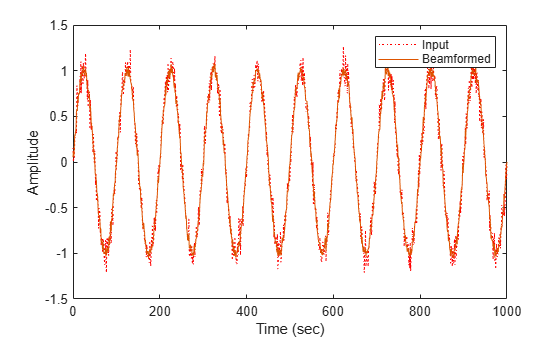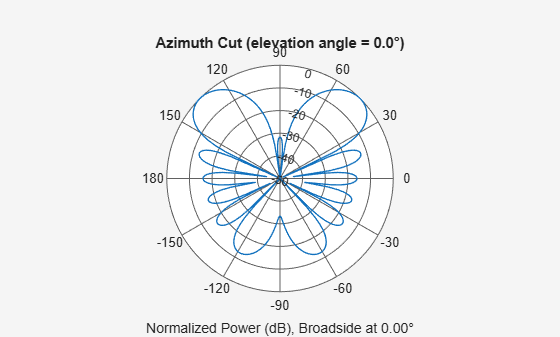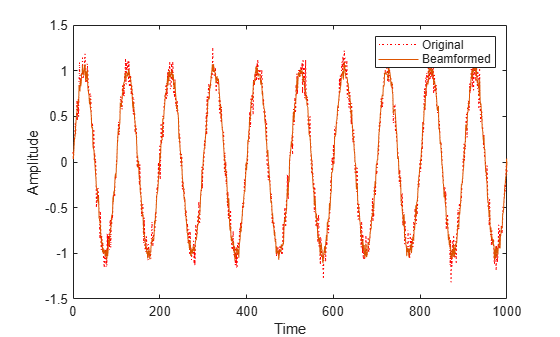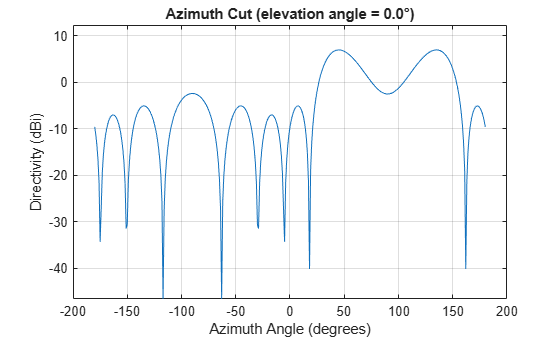phased.PhaseShiftBeamformer

Narrowband phase shift beamformer

Description

The phased.PhaseShiftBeamformer object implements a narrowband phase-shift beamformer. A phase-shift beamformer approximates a time-delay beamformer for narrowband signals by phase-shifting the arriving signal. A phase shift beamformer belongs to the family of conventional beamformers.

To beamform signals arriving at an array:

1. Create the phased.PhaseShiftBeamformer object and set its properties.

2. Call the object with arguments, as if it were a function.

Creation

Description

beamformer = phased.PhaseShiftBeamformer creates a phase-shift beamformer System object™, beamformer, with default property values.

beamformer = phased.PhaseShiftBeamformer(Name,Value) creates a phase-shift beamformer with each property Name set to a specified Value. You can specify additional name-value pair arguments in any order as (Name1,Value1,...,NameN,ValueN). Enclose each property name in single quotes.

Example: beamformer = phased.PhaseShiftBeamformer('SensorArray',phased.URA,'OperatingFrequency',300e6) sets the sensor array to a uniform rectangular array (URA) with default URA property values. The beamformer has an operating frequency of 300 MHz.

Properties

expand all

Unless otherwise indicated, properties are nontunable, which means you cannot change their values after calling the object. Objects lock when you call them, and the release function unlocks them.

If a property is tunable, you can change its value at any time.

Sensor array, specified as an array System object belonging to Phased Array System Toolbox. The sensor array can contain subarrays.

Example: phased.URA

Signal propagation speed, specified as a real-valued positive scalar. Units are in meters per second. The default propagation speed is the value returned by physconst('LightSpeed').

Example: 3e8

Data Types: single | double

Operating frequency, specified as a positive scalar. Units are in Hz.

Example: 1e9

Data Types: single | double

Source of beamforming direction, specified as 'Property' or 'Input port'. Specify whether the beamforming direction comes from the Direction property of this object or from the input argument, ANG. Values of this property are:

 'Property' Specify the beamforming direction using the Direction property. 'Input port' Specify the beamforming direction using the input argument, ANG.

Data Types: char

Beamforming directions, specified as a real-valued 2-by-1 vector or a real-valued 2-by-L matrix. For a matrix, each column specifies a different beamforming direction. Each column has the form [AzimuthAngle;ElevationAngle]. Azimuth angles must lie between –180° and 180° and elevation angles must lie between –90° and 90°. All angles are defined with respect to the local coordinate system of the array. Units are in degrees.

Example: [40;30]

Dependencies

To enable this property, set the DirectionSource property to 'Property'.

Data Types: single | double

The number of bits used to quantize the phase shift component of beamformer or steering vector weights, specified as a nonnegative integer. A value of zero indicates that no quantization is performed.

Example: 5

Data Types: single | double

If you set this property value to 'Distortionless', the gain in the beamforming direction is 0 dB. If you set this property value to 'Preserve power', the norm of the weights is unity.

Example: 'Preserve power'

Data Types: char

Enable the output of beamforming weights, specified as false or true. To obtain the beamforming weights, set this property to true and use the corresponding output argument, W. If you do not want to obtain the weights, set this property to false.

Data Types: logical

Usage

Description

example

Y = beamformer(X) performs phase-shift beamforming on the input signal, X, and returns the beamformed output in Y. To use this syntax, set DirectionSource to 'Property' and set the beamforming direction using the Direction property.

example

Y = beamformer(X,ANG) uses the ANG input argument to set the beamforming direction. To use this syntax, set the DirectionSource property to 'Input port'.

[Y,W] = beamformer(___) returns the beamforming weights, W. To use this syntax, set the WeightsOutputPort property to true.

Input Arguments

expand all

Input signal, specified as a complex-valued M-by-N matrix. If the sensor array contains subarrays, N is the number of subarrays; otherwise, N is the number of array elements.

The size of the first dimension of the input matrix can vary to simulate a changing signal length. A size change can occur, for example, in the case of a pulse waveform with variable pulse repetition frequency.

Data Types: single | double
Complex Number Support: Yes

Beamforming directions, specified as a real-valued 2-by-1 column vector, or 2-by-L matrix. L is the number of beamforming directions. Each column has the form [AzimuthAngle;ElevationAngle]. Units are in degrees. Each azimuth angle must lie between –180° and 180°, and each elevation angle must lie between –90° and 90°.

Example: [40;10]

Dependencies

To enable this argument, set the DirectionSource property to 'Input port'.

Data Types: single | double

Output Arguments

expand all

Beamformed output, returned as a complex-valued M-by-L matrix, where M is the number of rows of X and L is the number of beamforming directions.

Data Types: single | double
Complex Number Support: Yes

Beamforming weights, returned as a complex-valued N-by-L matrix. If the sensor array contains subarrays, N is the number of subarrays; otherwise, N is the number of elements. L is the number of beamforming directions.

Dependencies

To enable this output, set the DirectionSource property to true.

Data Types: single | double
Complex Number Support: Yes

Object Functions

To use an object function, specify the System object as the first input argument. For example, to release system resources of a System object named obj, use this syntax:

release(obj)

expand all

 step Run System object algorithm release Release resources and allow changes to System object property values and input characteristics reset Reset internal states of System object

Examples

collapse all

Apply phase-shift beamforming to a sinewave signal received by a 7-element ULA. The beamforming direction is 45° azimuth and 0° elevation. Assume the array operates at 300 MHz. Specify the beamforming direction using the Direction property.

Simulate the signal.

t = (0:1000)';
fsignal = 0.01;
x = sin(2*pi*fsignal*t);
c = physconst('Lightspeed');
fc = 300e6;
incidentAngle = [45;0];
array = phased.ULA('NumElements',7);
x = collectPlaneWave(array,x,incidentAngle,fc,c);
noise = 0.1*(randn(size(x)) + 1j*randn(size(x)));
rx = x + noise;

Set up a phase-shift beamformer and then beamform the input data.

beamformer = phased.PhaseShiftBeamformer('SensorArray',array,...
'OperatingFrequency',fc,'PropagationSpeed',c,...
'Direction',incidentAngle,'WeightsOutputPort',true);
[y,w] = beamformer(rx);

Plot the original signal at the middle element and the beamformed signal.

plot(t,real(rx(:,4)),'r:',t,real(y))
xlabel('Time (sec)')
ylabel('Amplitude')
legend('Input','Beamformed')Plot the array response pattern after applying the weights.

pattern(array,fc,[-180:180],0,'PropagationSpeed',c,'Type',...
'powerdb','CoordinateSystem','polar','Weights',w)Apply phase-shift beamforming to the signal received by a 5-element ULA. The beamforming direction is 45° azimuth and 0° elevation. Assume the array operates at 300 MHz. Specify the beamforming direction using an input port.

Simulate a sinewave signal arriving at the array.

t = (0:1000)';
fsignal = 0.01;
x = sin(2*pi*fsignal*t);
c = physconst('LightSpeed');
fc = 300e6;
incidentAngle = [45;0];
array = phased.ULA('NumElements',5);
x = collectPlaneWave(array,x,incidentAngle,fc,c);
noise = 0.1*(randn(size(x)) + 1j*randn(size(x)));
rx = x + noise;

Construct the phase-shift beamformer and then beamform the input data.

beamformer = phased.PhaseShiftBeamformer('SensorArray',array,...
'OperatingFrequency',fc,'PropagationSpeed',c,...
'DirectionSource','Input port','WeightsOutputPort',true);

Obtain the beamformed signal and the beamformer weights.

[y,w] = beamformer(rx,incidentAngle);

Plot the original signal at the middle element and the beamformed signal.

plot(t,real(rx(:,3)),'r:',t,real(y))
xlabel('Time')
ylabel('Amplitude')
legend('Original','Beamformed')Plot the array response pattern after applying the weights.

pattern(array,fc,[-180:180],0,'PropagationSpeed',c,'CoordinateSystem','rectangular','Weights',w)Algorithms

expand all

 Van Trees, H.L. Optimum Array Processing. New York, NY: Wiley-Interscience, 2002.

 Johnson, Don H. and D. Dudgeon. Array Signal Processing. Englewood Cliffs, NJ: Prentice Hall, 1993.

 Van Veen, B.D. and K. M. Buckley. “Beamforming: A versatile approach to spatial filtering”. IEEE ASSP Magazine, Vol. 5 No. 2 pp. 4–24.